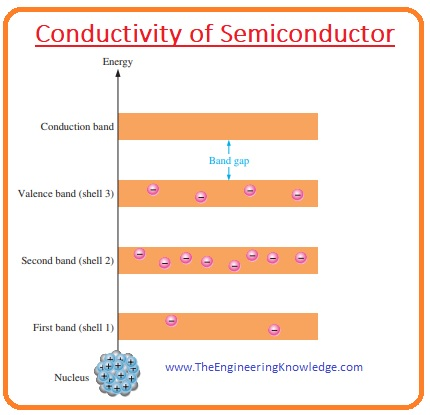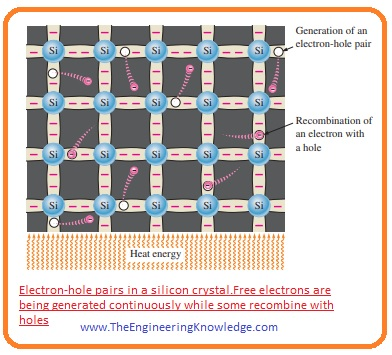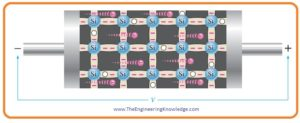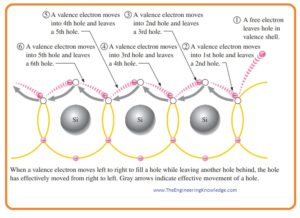Hello, fellows, I hope all of you are having fun in your life. In today’s tutorial, we will discuss the Conductivity of semiconductors.  The number of electrons present in any substance defines the conductance of that material. The element that shows a high value of conductance have large numbers of electrons such as conductors. While the material has less or zeroes free electrons also have less value of conductance and called insulators.

In semiconductors, the number of free electrons is between conductors and insulator so there conductivity is also between conductors and insulators. In today’s post, we will have a detailed look at the conductance behavior of semiconductors and will explain which factors play roles for conductance in these materials. So let’s get started with the Conductivity of Semiconductor.

#### Conductivity of Semiconductor

• As we know in every atom there are certain shells or energy levels each shell has specified numbers of electrons.
• Every shell is a certain distance from their nearing shell this gap among two shells is called the band gap and there are no electrons reside in this band.
• In the below figure, you can see the energy diagram of pure silicon atom that is in a non-excited state and there are zero electrons in energy band.
• Such a state exits at a 0-K temperature that is absolute temperature.

#### Conduction Electrons and Holes

• In pure or intrinsic silicon material at normal temperature outermost or valence shell electrons have enough amount of energy to cross the band gap and move to the conduction band.
• The electron after removal from the shell is called conduction electrons.
• This arrangement is shown in this given figure.• After the movement of an electron from valence shell to conduction band, there is free space created that called
• Each electron moved from valence shell to conduction band there is a hole created and also make electron-hole pair at the valence shell.
• If an electron in conduction band losses energy then came back to free space created after moving to the conduction band.
• This phenomenon of creation of holes and electron pairs is shown in a given figure.#### Electron and Hole Current

• If we apply power source to the pure silicon crystal then electrons in shells will gain energy and move to the conduction band.• These free electrons will move to the positive terminal of battery this movement of electrons in semiconductor substance is called electron current.
• When electron left their respective place hole created due to these hole creation other current flows in substance.
• Some electrons in valence shells that not have a high value of energy not move to the conduction band but they can move to their adjacent hole created by the high energy electron.
• When an electron move hole is created in its place that way holes also moved and current will flow due to these holes.
• This configuration is shown in a given figure.• In valence electrons, current flows due to the holes called hole current.
• So we can conclude that current in semiconductors is flow due to the free electrons and holes, these holes are space created by the moving electrons in opposite directions.
• It is very informative to compare the current movement in conductor and semiconductors that in semiconductors the current flow due to the 2 charges while in conductor current flows due to one type of charges like in copper.
• The atoms in copper make a different category of crystal in which the atoms do not have a covalent bond with one but comprise numerous positive ions.

That is a complete post on the Conductivity of Semiconductor if you have any questions ask in comments. See you in the next tutorial.